# Chapter 4: Scaling and Translation

by Kirby Urner
First posted: Oct 29, 1998
Last modified: Nov 23, 1998

To scale a shape is to change its size. If the scale factor is greater than 1, the shape will grow. A scale factor value less than 1, but still positive, will cause it to shrink.

Internally, the scale() method in our polyhedron class passes a scale factor to oMatrixOps, where it gets used as a multiplier against all coordinates in the shape's Points table.

Additionally, to scale a shape is to changes its volume by a third power of the scale factor.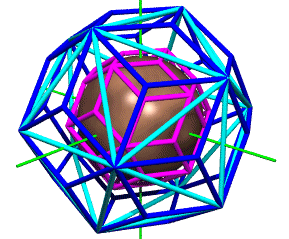Our subclassed polyhedra all start with some defined shapevolume property, which gets multiplied by scalefactor^3 in the scale() method. The large blue 30-faced rhombic triacontahedron in the above figure has a body- to face-center radius of phi (about 1.618) and a volume of about 21.21. The inscribed 30-edged icosahedron (cyan) is a little smaller, weighing in at about 18.51:

```define class icosahedron as polyhedron
shapeid = "I1"
snapshot = "I1points"
shapevolume = 18.51229586821915
shapecolor = "Cyan"
enddefine
```

The smaller rhombic triacontahedron (magenta), shrink-wrapped around a unit-radius sphere, has a volume of precisely 5 -- a result of internalized scaling properties designed for this purpose. Its body- to face-center radius is a hair less than the sphere's:

```define class rhtriac as polyhedron
shapeid = "RT1"
snapshot = "RT1points"
shapevolume = 20 * (9/8)^0.5  && 20 * (synergetics constant)^3
emodfactor = 2/(1+5^.5) && 1/phi
tmodfactor = ((2^.5 * (2 + 5^.5))/6)^(1/3) && E-mod -> T-mod Rh Triac
shapecolor = "Magenta"
enddefine
```

The script below invokes these internal scale factors to generate the VRML view at right. This script is essentially the same as the one used to create the Povray script behind the above ray tracing, except in this case the WriteVRML subclass of WritePoly was used, instead of the WritePov subclass:

 ```procedure rhtriac orhtriac = createobject("rhtriac") oIcosa = createobject("Icosahedron") oSph = createobject("sphere") oWriteVRML = createobject("WriteVRML") oWriteVRML.writeoutput(orhtriac) oWriteVRML.writeoutput(oIcosa) orhtriac.scale(orhtriac.emodfactor) orhtriac.scale(orhtriac.tmodfactor) oWriteVRML.writeoutput(orhtriac) oWriteVRML.makeaxes() oWriteVRML.writeoutput(oSph) return ```click for VRML view (if plug-in installed)
 To translate a shape is to slide it along some arrow or vector, passed to the translate() method in either xyz or quadray format. For example, the 12 permutations of {2,1,1,0} (all combinations of one 2, two 1s and a 0) point to the 12 corners of the cuboctahedron of volume 20. These are also the loci for unit-radius sphere centers closest-packed around a nuclear sphere in a ccp (= fcc = ivm) arrangement. To illustrate this fact, and to highlight the role of the 6-volumed rhombic dodecahedron as a space-filling "cage" for closest-packed unit-radius spheres, we will slide these shapes along two of these quadray-specified vectors. We'll throw in a cuboctahedron (in yellow) as well. The procedure for generating a corresponding VRML view is the same, except for the subclass of WritePoly used:
 ```procedure rhdodecas * create objects oRD = createobject("rhdodeca") oCubocta = createobject("cubocta") oSph = createobject("sphere") oWritePOV = createobject("WritePOV") oWritePOV.makeaxes() * draw shapes around the origin oWritePOV.writeoutput(oRD) oWritePOV.writeoutput(oSph) oWritePOV.writeoutput(oCubocta) * translate rh dodeca and sphere * and display oRD.translate(2,1,1,0) oSph.translate(2,1,1,0) oWritePOV.writeoutput(oRD) oWritePOV.writeoutput(oSph) * move them home, translate to another * corner of the cubocta, and display again oRD.gohome() oSph.gohome() oRD.translate(2,1,0,1) oSph.translate(2,1,0,1) oWritePOV.writeoutput(oRD) oWritePOV.writeoutput(oSph) return ```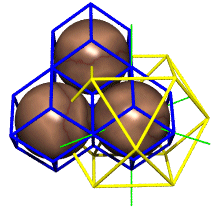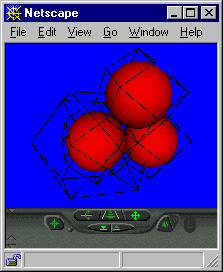click for VRML view (if plug-in installed) Now that we have a translate() method, we have the means to enhance our rotate() method as per our suggestion in Chapter 1. To "rotate in place", we keep track of an object's "pivot point" (usually its body center). This pivot point was added to our polyhedron class as a new property, and to our new sphere class (spheres translate and scale, but don't meaningfully rotate in any sense that would affect our displays). To use the rotation matrices already defined, we translate a shape to the origin, rotate it, and return it to its original location. For example, the script below defines two tetrahedra, slides one of them along the X axis, and then rotates it in place by 90 degrees. Note the "speckled" appearance of their shared edge -- a result of both black and orange cylinders drawing in the same space. Here's the VFP script:
 ```procedure testrotate oTetra1 = createobject("tetrahedron") oTetra2 = createobject("tetrahedron") oWritePOV = createobject("WritePOV") oWritePOV.makeaxes() oWritePOV.writeoutput(oTetra1) oTetra2.translate(2^.5,0,0) oTetra2.shapecolor="Black" oTetra2.rotate("X",90) oWritePOV.writeoutput(oTetra2) return ```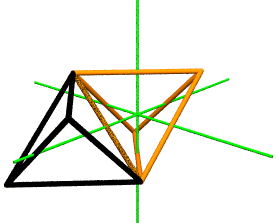On-line Resources: Chapters 1, 2, 3, 5, 6 and 7 of this webtext. Source code: polyclasses.prg (class defs), main.prg (scripts) -- Ver. 1.0 Zip file containing source code, sample output files -- Ver. 1.0 Oct 29 1998 Beyond Flatland: Geometry for the 21st Century Introducing the Concentric Hierarchy Return to Symbols and Operators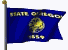Oregon Curriculum Network